﻿ Weighted average maturity: What is it, Working, Calculation, FAQ | POEMS

# Weighted average maturity

## Weighted average maturity

For pools of mortgage-backed securities, the weighted average maturity is often a more widely used indicator of maturity. It computes an average maturity period for each security in a debt portfolio, weighted in accordance with the portfolio’s dollar investment. The sensitivity to changes in interest rates is greater in portfolios with a higher weighted average maturity.

By understanding the weighted average maturity of their portfolio, investors can make informed decisions about when to buy and sell bonds. This can help them to avoid losses in the event of a market downturn.

## What is weighted average maturity?

A weighted average maturity is the average of the maturities of a group of securities, with each security’s weight in the averaging process being proportional to its market value.

The higher the average maturity, the longer it takes for each portfolio item to mature. The weighted average maturity is utilized to manage debt portfolios and evaluate the effectiveness of debt portfolio managers.

## Understanding weighted average maturity

When it comes to investments, the weighted average maturity is an important metric to understand. Simply put, the weighted average maturity or WAM measures the average length of time until the maturity of the bonds in a portfolio. The weighting part of the equation refers to the fact that bonds with longer maturities are given more weight, or importance, in the calculation.

Why is the WAM important? Well, it can give investors a good idea of the riskiness of a portfolio. For example, a portfolio with a WAM of 10 years will be more sensitive to interest rate changes than a portfolio with a WAM of 5 years. That’s because bonds with longer maturities are more susceptible to interest rate changes than bonds with shorter maturities.

Investors can use the WAM to decide how to allocate their assets. For example, an investor looking for a more conservative portfolio might choose to invest in bonds with shorter maturities. In comparison, an investor looking for a more aggressive portfolio might decide to invest in bonds with longer maturities.

Of course, the WAM is just one metric investors should consider when making investment decisions. But it is a helpful tool for understanding the riskiness of a portfolio and for making asset allocation decisions.

## How does weighted average maturity work?

The WAM is used to give investors an idea of the riskiness of a bond portfolio. A higher WAM means that the portfolio is riskier.

## How is weighted average maturity calculated?

First, determine the weight of each security in the portfolio to calculate the average weighted maturity. The weight is determined by the security’s market value divided by the portfolio’s total market value.

Once the weights are determined, calculate the maturity of each security. The maturity is the number of years until the security matures. To get the weighted maturity, multiply the weight of the security by the maturity.

Finally, add the weighted maturity of all the securities in the portfolio to get the average weighted maturity.

For example, let’s say you wish to calculate the WAM of three bonds,

Bond X is \$2,000, which matures in 10 years.

Bond Y is \$4,000, which matures in 8 years.

Bond Z is \$8,000, which matures in 4 years.

 Bond Amount Weight Maturity Weighted Maturity Bond X \$2,000 14.3% 10 1.43 Bond Y \$4,000 28.6% 8 2.29 Bond Z \$8,000 57.1% 4 2.28 \$14,000 100% 6

Therefore, the weighted average maturity period for the bond portfolio is 6 years.

## What does weighted average maturity signify?

Weighted average maturity is a measure of a bond fund’s sensitivity to changes in interest rates. It is calculated by weighting the maturity of each bond in the fund by its percentage of the fund’s total assets. A result is a single number representing the average maturity of the bonds in the fund.

A bond fund with a higher weighted average maturity will be more sensitive to changes in interest rates than a fund with a lower weighted average maturity. For example, if interest rates rise, the value of a bond fund with a higher weighted average maturity will fall more than that of a fund with a lower weighted average maturity.

Weighted average maturity can be a helpful tool for investors trying to manage their exposure to interest rate risk. For example, an investor concerned about the potential for rising interest rates may choose to invest in a bond fund with a lower weighted average maturity.

Weighted average maturity bonds are bonds whose coupon payments are weighted according to the time remaining until maturity. The weights are usually based on the number of days remaining until maturity. The advantage of weighted average maturity bonds is that they provide a measure of protection against interest rate changes.

A good weighted average maturity is weighted according to the importance of the different maturities. For example, if short-term maturities are more critical, they should be given greater weight. The weighted average maturity can be used to measure the risk of a portfolio’s risk and the average return.

A weighted average maturity calculator is a tool that can be used to calculate the weighted average maturity of a portfolio of bonds. The calculator takes into account the different maturities of the bonds in the portfolio, as well as the different weights that are assigned to each bond. This information is then used to calculate the portfolio’s weighted average maturity.

The weighted average maturity of assets is a measure of the average maturity of all the assets in a portfolio, weighted according to their respective market values.

This metric helps assess the riskiness of a portfolio, as assets with longer maturities are typically more sensitive to interest rate changes than those with shorter maturities.

The weighted average term of maturity of a debt security is the average length of time until the debt instrument matures, weighted by the outstanding principal amount of each security. This calculation is used to give investors an idea of the average maturity of the debt in the portfolio.

## Category

### Read the Latest Market Journal#### Top traded counters in August 2023

Published on Sep 19, 2023 157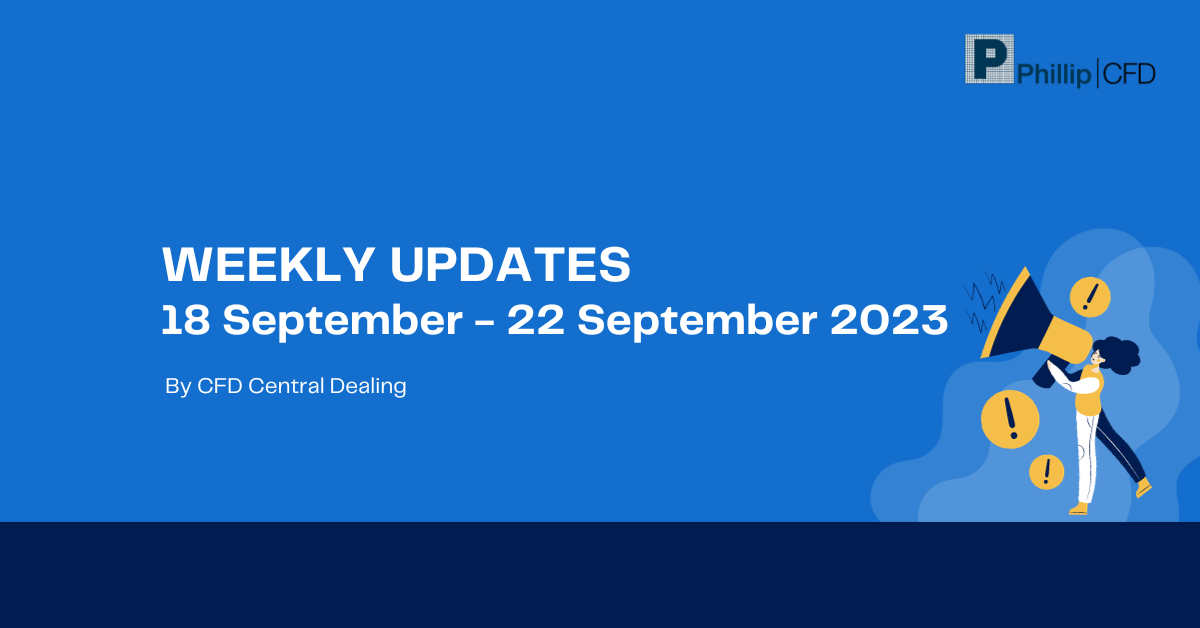#### Weekly Updates 18/9/23 – 22/9/23

Published on Sep 18, 2023 25

This weekly update is designed to help you stay informed and relate economic and company...#### The Merits of Dollar Cost Averaging

Published on Sep 15, 2023 42

Have you ever seen your colleagues, friends or family members on the phone with their...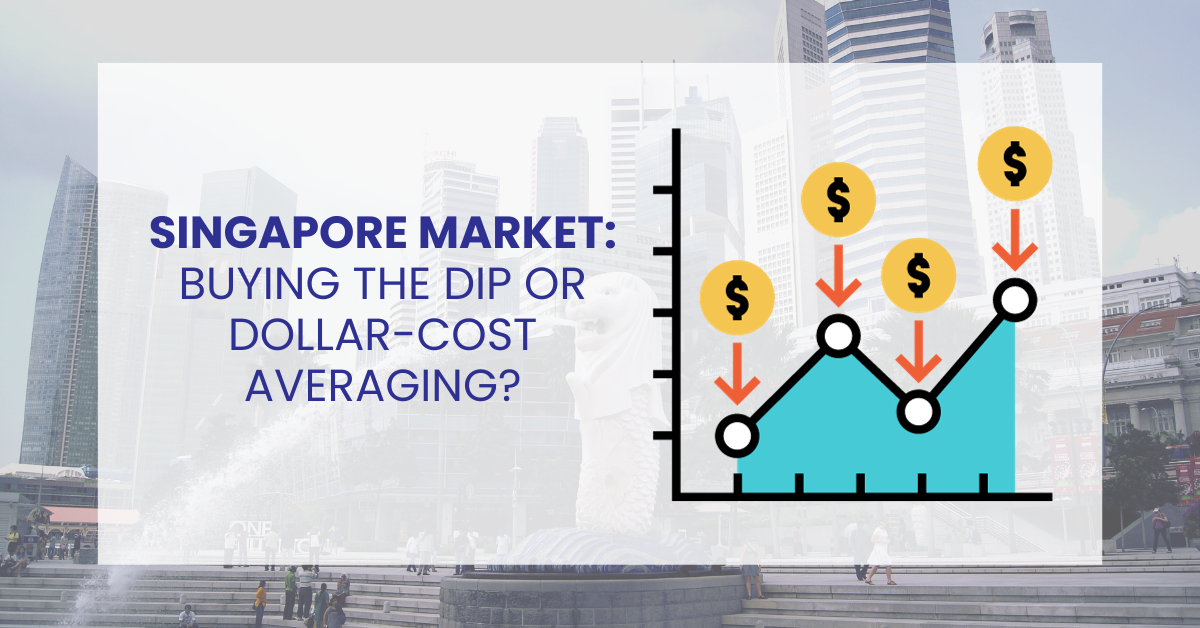#### Singapore Market: Buy the Dip or Dollar-Cost Averaging?

Published on Sep 14, 2023 46

To the uninitiated, investing in the stock market can be deemed exhilarating and challenging. The...#### What are covered calls and why are they so popular?

Published on Sep 12, 2023 350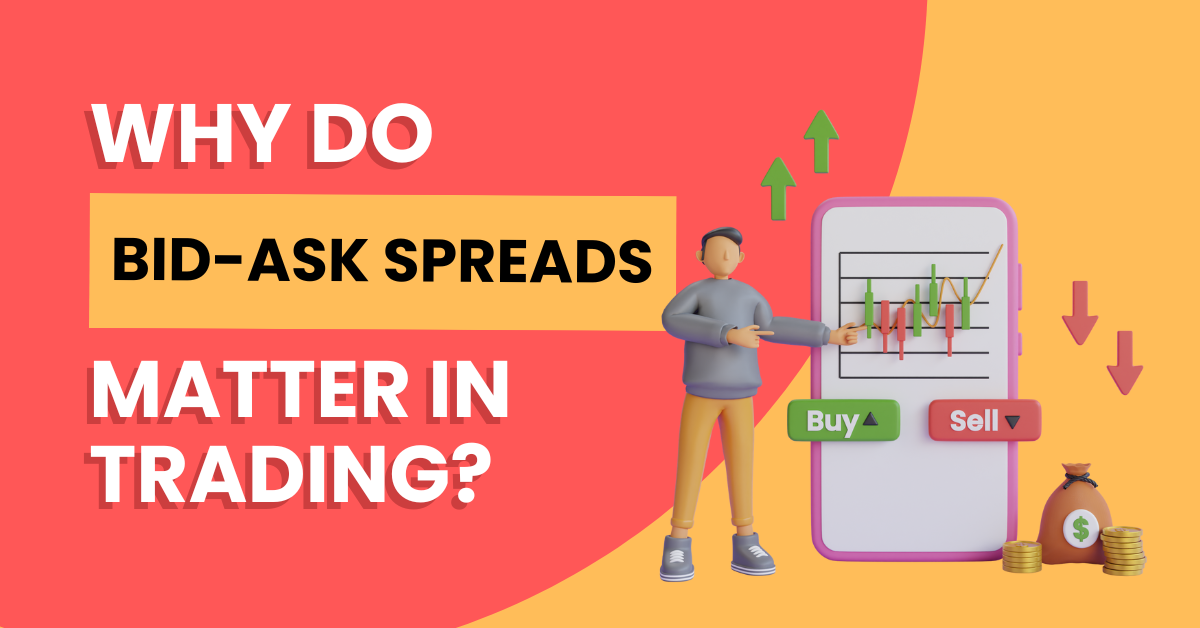Published on Sep 11, 2023 30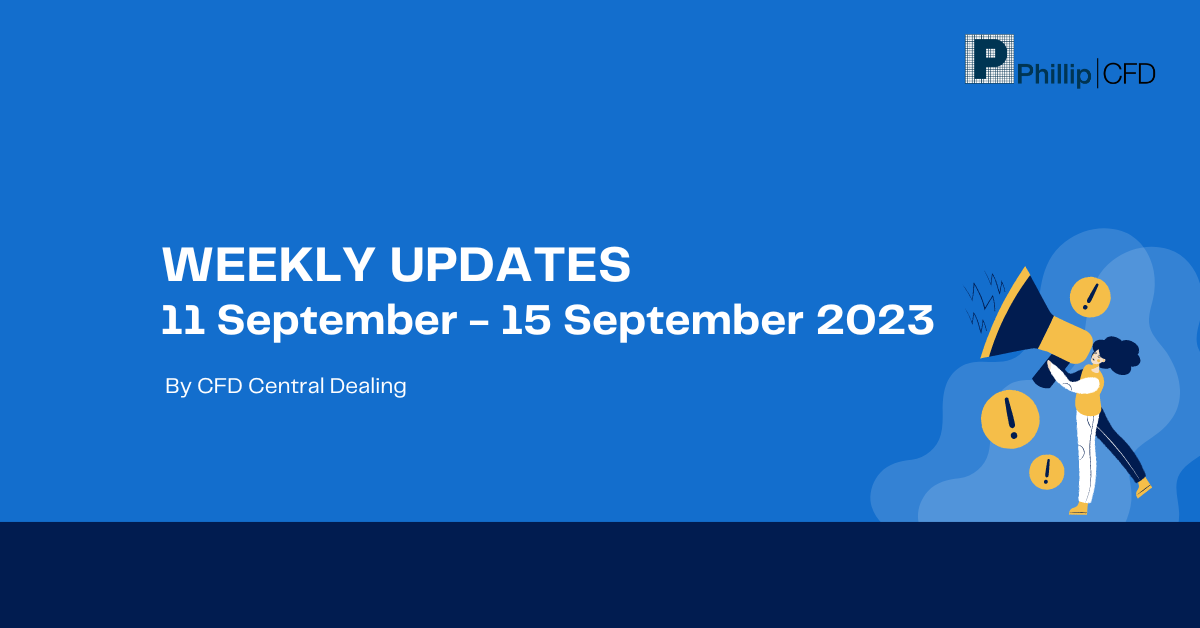#### Weekly Updates 11/9/23 – 15/9/23

Published on Sep 11, 2023 13

This weekly update is designed to help you stay informed and relate economic and company...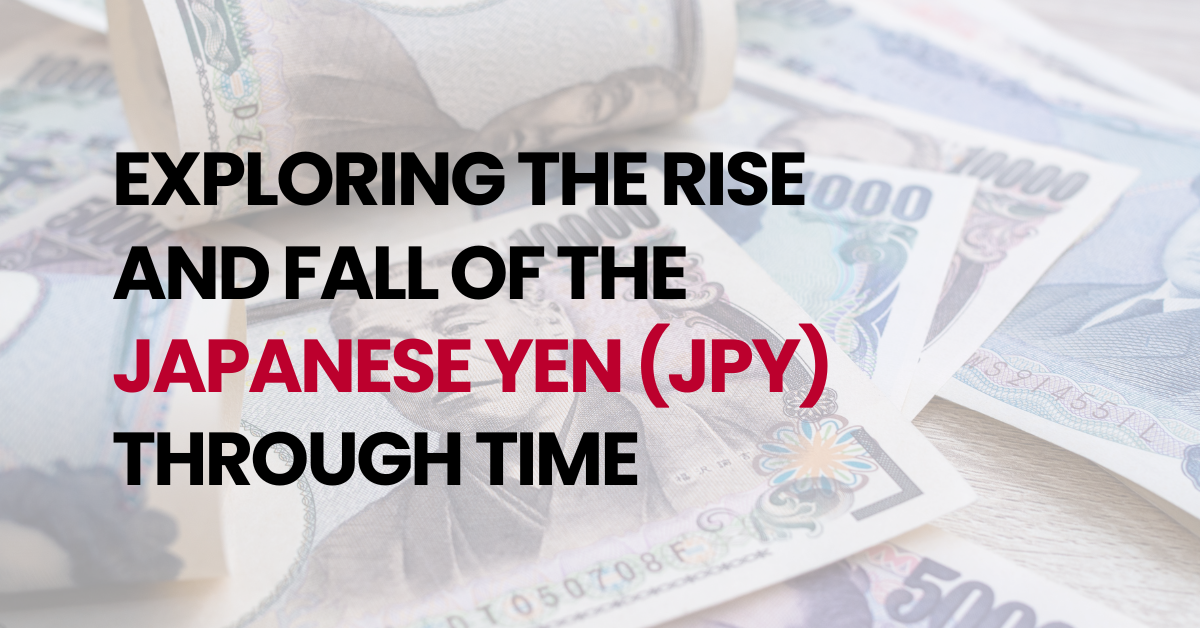#### Exploring the Rise and Fall of the Japanese Yen (JPY) Through Time

Published on Sep 5, 2023 80

The Japanese Yen (JPY) has long been considered a safe-haven currency due to Japan’s strong...